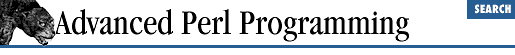Chapter 6Modules6.6 Nesting Packages

Since all packages are global in scope, nesting of packages is not supported. However, you can have two packages, one called A and another called A::B, to give an illusion of nesting. This is a naming convention only and implies no necessary relation between the two packages; however, this convention is typically applied to groups of related packages, and the term "nested packages" does not seem wrong in such cases. For example, you could have a module called Math::Matrix for matrix manipulation and another called Math::Poisson that supports an infrastructure for simulating queuing models. The only relation between the two modules is that they both are mathematical in nature; they don't share any implementation characteristics.

The :: notation is used as before to access variables and subroutines of nested packages:

\$p = Math::Poisson::calculate_probability(\$lambda, \$t);
print \$Math::Constants::PI;

When you say use File, recall that Perl looks for a file called File.pm. If you say, use Math::Poisson, Perl looks for a file called Math/Poisson.pm (directory Math, file Poisson.pm). The double colon gets translated to a filename separator, because the colon has a special significance for DOS filenames. Perl imposes no limits on the level of nesting.